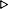ToC DocOverview CGDoc RelNotes Index PermutedIndex
Allegro CLANSI Common Lisp12 Numbers12.2 Dictionary of Numbers

 12.2.62 logand, logandc1, logandc2, logeqv, logior, lognand, lognor, lognot, logorc1, logorc2, logxor Function

Syntax:
logand &rest integersresult-integer

logandc1 integer-1 integer-2result-integer

logandc2 integer-1 integer-2result-integer

logeqv &rest integersresult-integer

logior &rest integersresult-integer

lognand integer-1 integer-2result-integer

lognor integer-1 integer-2result-integer

lognot integerresult-integer

logorc1 integer-1 integer-2result-integer

logorc2 integer-1 integer-2result-integer

logxor &rest integersresult-integer

Arguments and Values:
integers - integers.

integer - an integer.

integer-1 - an integer.

integer-2 - an integer.

result-integer - an integer.

Description:
The functions logandc1, logandc2, logand, logeqv, logior, lognand, lognor, lognot, logorc1, logorc2, and logxor perform bit-wise logical operations on their arguments, that are treated as if they were binary.

The next figure lists the meaning of each of the functions. Where an `identity' is shown, it indicates the value yielded by the function when no arguments are supplied.

Bit-wise Logical Operations on Integers
Function Identity Operation performed
logandc1 - and complement of integer-1 with integer-2
logandc2 - and integer-1 with complement of integer-2
logand -1 and
logeqv -1 equivalence (exclusive nor)
logior 0 inclusive or
lognand - complement of integer-1 and integer-2
lognor - complement of integer-1 or integer-2
lognot - complement
logorc1 - or complement of integer-1 with integer-2
logorc2 - or integer-1 with complement of integer-2
logxor 0 exclusive or

Negative integers are treated as if they were in two's-complement notation.

Examples:
(logior 1 2 4 8)15
(logxor 1 3 7 15)10
(logeqv)-1
(logand 16 31)16
(lognot 0)-1
(lognot 1)-2
(lognot -1)0
(lognot (1+ (lognot 1000)))999

;;; In the following example, m is a mask.  For each bit in
;;; the mask that is a 1, the corresponding bits in x and y are
;;; exchanged.  For each bit in the mask that is a 0, the
;;; corresponding bits of x and y are left unchanged.
(flet ((show (m x y)
(format t "~%m = #o~6,'0O~%x = #o~6,'0O~%y = #o~6,'0O~%"
m x y)))
(let ((m #o007750)
(x #o452576)
(y #o317407))
(show m x y)
(let ((z (logand (logxor x y) m)))
(setq x (logxor z x))
(setq y (logxor z y))
(show m x y))))m = #o007750x = #o452576y = #o317407m = #o007750x = #o457426y = #o312557NIL

Exceptional Situations:
Should signal type-error if any argument is not an integer.

boole

Notes:
(logbitp k -1) returns true for all values of k.

Because the following functions are not associative, they take exactly two arguments rather than any number of arguments.

(lognand n1 n2) ==(lognot (logand n1 n2))
(lognor n1 n2) ==(lognot (logior n1 n2))
(logandc1 n1 n2) ==(logand (lognot n1) n2)
(logandc2 n1 n2) ==(logand n1 (lognot n2))
(logiorc1 n1 n2) ==(logior (lognot n1) n2)
(logiorc2 n1 n2) ==(logior n1 (lognot n2))
(logbitp j (lognot x)) ==(not (logbitp j x))

Allegro CL Implementation Details:
None.© Franz Inc. 1998-2019 - File last updated 02-07-2019